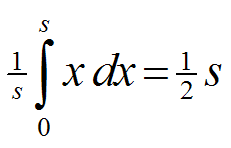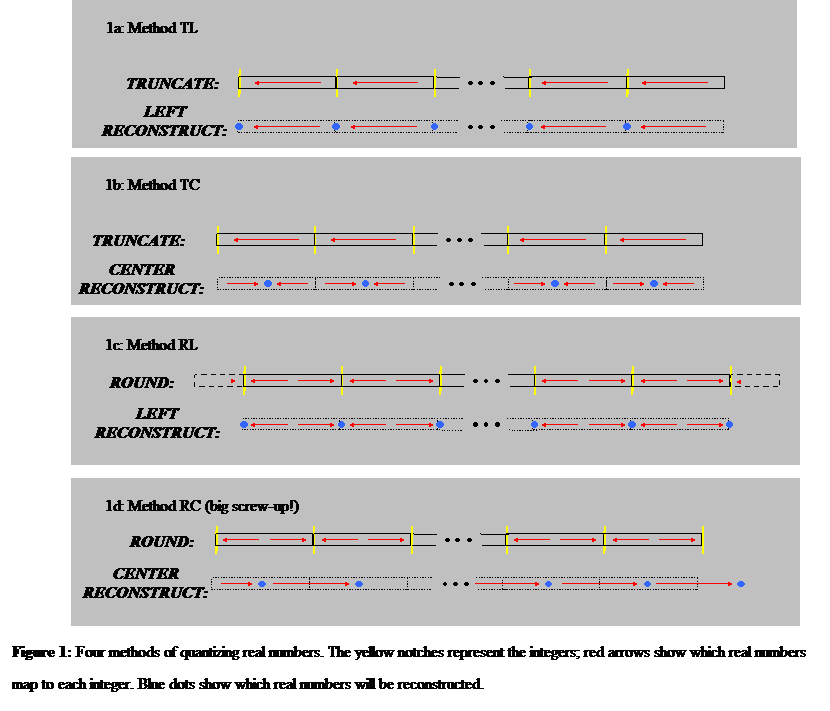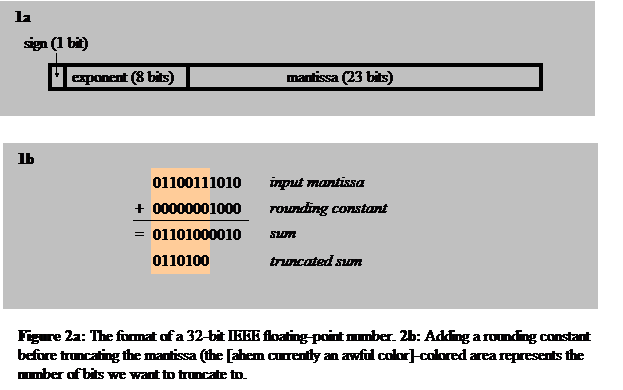The Inner Product, June 2002
Jonathan Blow (jon@number-none.com)
Last updated 12 January 2004

Scalar Quantization

Related articles:

Packing Integers (May 2002)
Transmitting Vectors (July 2002)

Arithmetic Coding, Part 1 (August 2003)
Arithmetic Coding, Part 2 (September 2003)

Adaptive Compression Across Unreliable Networks (October 2003)
Piecewise Linear Data Models (November 2003)

Last month I showed some methods of packing integers together.  The purpose of that was to fit things in a small space for save-games, or to save bandwidth for online games (massively multiplayer games spend a lot of money on bandwidth!)  This month we'll extend our methods to handle floating-point values.  We'll do this by carefully converting these scalars into integers, and then packing the integers as described last month.

We want to map a continuous set of real numbers onto a set of integers, a process known as quantization.  We quantize by splitting the set into a bunch of intervals, and assigning one integer value to each interval.  When it's time to decode, we map eac integer back to a scalar value that represents the interval.  This scalar will not be the same as the input value, but if we're careful it will be close.  So our encoding here is lossy.

Game programmers often perform this general sort of task.  If they're not being cautious and thoughtful, they'll likely do something like this:

// 'f' is a float between 0 and 1

const int NSTEPS = 64;

int encoded = (int)(f * NSTEPS);

if (encoded > NSTEPS - 1) encoded = NSTEPS - 1;

Then they decode like this:

const float STEP_SIZE = (1.0f / NSTEPS);

float decoded = encoded * STEP_SIZE;

I am going to name these two pieces of code, because I want to talk about what's wrong with them and suggest alternatives.  The first piece of code multiplies the input by a scaling factor, then truncates the fractional part of the result (the cast to int implicitly performs the truncation).  I am going to call this block of code 'T', for Truncate.

The second piece of code recovers a floating-point value by scaling the encoded integer value.  I will call it 'L' for Left Reconstruction -- it gives us the value of the left-hand side of each interval (Figure 1a).  Using these two steps together gives us the 'TL' method of encoding and decoding real numbers.

As shown in Figure 1a, the net result of performing the TL process on input values is to shunt them to the left-hand side of whichever interval they started in.  (If you don't see this right away, just keep playing with some example input values until you get it).  This leftward motion is bad for most applications, for two main reasons.  The first problem is that our values will in general shift toward zero, which means that there is a bias toward energy loss.  The second problem is that we end up wasting storage space (or bandwidth).  To see why, let's look at an alternative.

I am going to replace the 'L' part of TL with a different reconstruction method, which is mostly the same except that it adds an extra half-interval size to the output value.  As a result, it shunts input values to the center of the interval they started in, as shown in Figure 1b.  We'll call it 'C' for Center Reconstruction, and here is the source code:

const float STEP_SIZE = (1.0f / NSTEPS);

float decoded = (encoded + 0.5f) * STEP_SIZE;

When we use this together with the same truncation encoder as before, we get the codec TC.  We can see from the diagram that TC will increase some inputs and decrease others.  If we encode many random values, the average output value converges to the average input value, meaning there is no change in total energy.

Now let's think about bandwidth.  The amount of storage space we use is determined by the range of integers we reserve for encoding our real-numbered inputs (the value of NSTEPS in the code snippets above).  In order to find an appropriate value for NSTEPS, we need to choose a maximum error threshold by which our input can be perturbed.

When we use TL to store and retrieve arbitrary values, the mean displacement (the difference between the output and the input) iswhere s is 1/NSTEPS.  When we use TC, the mean displacement is only 1/4 s.  Thus, to meet a given error target, NSTEPS has to be twice as high with TL as it does with TC.  So TL needs to spend an extra bit of information to achieve the same guaranteed accuracy that TC gets naturally.Though TC is a step above TL in many ways, it has a property that can be problematic: there's no way to represent zero.  When you feed it zero, you get back a small positive number.  If you're using this to represent an object's speed, then whenever you save and load a stationary object, you'll find it slowly creeping across the world.  That's really no good.

We can fix this by going back to left-reconstruction, but then, instead of truncating the input values downward, we round them to the nearest integer.  We'll call this 'R' for rounding.  Being seasoned programmers we know that you round a nonnegative number by adding 1/2 and then truncating it.  Thus the source code is:

// 'f' is a float between 0 and 1

const int NSTEPS = 64;

int result = (int)(input * (NSTEPS - 1) + 0.5f);

if (result > NSTEPS - 1) result = NSTEPS - 1;

The method RL is shown in Figure 1c.  It's got the same set of output values that TL has, but it maps different inputs to those outputs.  RL has the same mean error as TC, which is good.  It can store and retrieve 0 and 1; 1 is important since, if you're storing something representing 'fullness' (like health or fuel or something), you want to be able to say that the value is at 100%.

It's nice to be able to represent the endpoints of our input range, but we do pay a price for that: RL is less bandwidth-efficient than TC.  Note that I changed the scaling factor from NSTEPS, as 'T' uses, to NSTEPS - 1.  This allows values near the top of the input range to be mapped to 1.  If I hadn't done this, values near 1 would get mapped further downward than the other numbers in the input range, and thus would introduce more error than we'd like.  Also, RL's average error would have been higher than TC's, and it would have regained a slight tendency toward energy decrease.  I avoided this badness by permitting the half-interval near 1 to map to 1.

But this costs bandwidth.  Notice that the half-intervals at the low and high ends of the input range only cover one interval's worth of space, put together.  So we're wasting one interval's worth of information, made of the pieces that lie outside the edges of our input.  If an RL codec uses n different intervals, each interval will be the same size as it would be in a TC codec with n-1 pieces.  So to achieve the same error target as TC, RL needs one extra interval.

If our value of NSTEPS was already pretty high, then adding 1 to it is not a big deal; the extra cost is low.  But if NSTEPS is a small number, the increment starts to be noticable.  You'll want to choose between TC and RL based on your particular situation.  RL is the safest, most robust thing to use by default in cases where you don't want to think too hard.

Don't Do Both

Once, when I was on a project that wasn't thinking clearly about this stuff, we used a method RC, which both rounded and center-reconstructed.  This unfortunate choice is shown as Figure 1d.  It is arguably worse than the TL that we started with, because it generally increases energy.  Whereas decreasing energy tends to damp a system stably, increasing energy tends to make systems blow up.  In this particular project, we thought we were being careful about rounding; but we didn't do enough observation to see that the two rounding steps cancel each other out.  Live and learn.

Interval Width

So far we've been assuming that the intervals should all be the same size.  It turns out that this is optimal when your input vales all occur with equal probability.  Since we're not talking about probability modeling today, we'll just keep on assuming intervals of equal size (this approach will lend us significant clarity next month, when we deal with multidimensional quantities).  But you might imagine that, if you knew most of your values landed in one spot of the input domain, it would be better to have smaller intervals there, and larger ones elsewhere.  You'd probably be right, depending on your application's requirements.

Varying Precision

So far, we've talked about a scheme of encoding real numbers that's applicable when you want error of constant magnitude across the range of inputs.  But sometimes you want to adjust the absolute error based on the magnitude of the number.  For example, the whole idea of floating-point numbers is that the absolute error rises as the number gets bigger.  This is useful because often what you care about is the ratio of magnitudes between the error and the original quantity.  So with floating point, you can talk about numbers that are extremely large, as long as you can accept proportionally rising error magnitudes.

One way to implement such varying precision would be to split up our input range into intervals that get bigger as we move toward the right.  But in the interest of expediency, I am going to adopt a more hacker-centric view here.  Most computers we deal with already store numbers in IEEE floating-point formats, so a lot of work is already done for us.  If we want to save those numbers into a smaller space, we can chop the IEEE representation apart, reduce the individual components, and pack them back together into something smaller.

IEEE floating point numbers are stored in three parts: a sign bit s, an exponent e, and a mantissa m.  Their layout in memory is shown in Figure 2a.  The real number represented by a particular IEEE float is -1^s m x 2^m.  The main trick to remember is that the mantissa has an implicit '1' bit tacked onto its front.  There are plenty of sources available for reading about IEEE floating point, so I'll leave it at that.  There are some references in For Further Information.

If we know that we're only processing nonnegative numbers, we can do away with the sign bit.  The 32-bit IEEE format provides 8 bits of exponent, which is probably more than we want.  And then, of course, we can take an axe to the mantissa, lowering the precision of the number.

Our compacted exponent does not have to be a power of two, and does not even have to be symmetric about 0 like the IEEE's is.  We can say "I want to store exponents from -8 to 2", and use the Multiplication_Packer from last month to store that range.  Likewise, we could chop the mantissa down to land within an arbitrary integer range.  To keep things simple, though, we will restrict our mantissa to being an integer number of bits.  This will make rounding easier.

To make the mantissa smaller, we round, then truncate.  To round the mantissa, we want to add .5 scaled such that it lands just below the place we are going to cut (Figure 2b).  If the mantissa consists of all '1' bits, adding our rounding factor will cause a carry that percolates to the top of the mantissa.  So we need to detect this, which can easily be done if the mantissa is being stored in its own integer (we just check the bit above the highest mantissa bit, and see if it has flipped to 1).  If that bit has flipped, we increment the exponent.

Rounding the mantissa is important.  Just as in the quantization case, if we don't round the mantissa, then we need to spend an extra bit worth of space to achieve the same accuracy level, and we have net energy decrease, etc.Sample Code

This month's sample code implements the TC and RL forms of quantization.  It also contains a class called Float_Packer; you tell the Float_Packer how large a mantissa and exponent to use, and whether you want a sign bit.